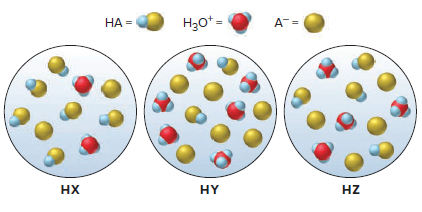# Problem: The following scene represents three weak acid HA (where A = X, Y, or Z) dissolved in water (H2O is not shown):(e) If equimolar amounts of the sodium salts of the acids (NaX, NaY, and NaZ) were dissolved in water, which solution would have the highest pOH? The lowest pH?

###### FREE Expert Solution

The least acidic (based on the number of H3O+) is HX.

Recall:
$\overline{){\mathbf{pH}}{\mathbf{=}}{\mathbf{-}}{\mathbf{log}}\mathbf{\left[}{\mathbf{H}}_{\mathbf{3}}{\mathbf{O}}^{\mathbf{+}}\mathbf{\right]}}$

pH and pOH are related through this equation:

80% (234 ratings)###### Problem Details

The following scene represents three weak acid HA (where A = X, Y, or Z) dissolved in water (H2O is not shown):

(e) If equimolar amounts of the sodium salts of the acids (NaX, NaY, and NaZ) were dissolved in water, which solution would have the highest pOH? The lowest pH?